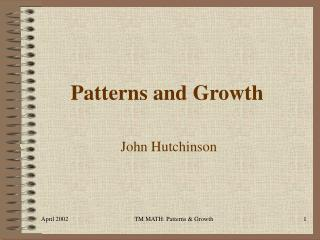DownloadDownload PresentationPatterns and Growth

# Patterns and Growth

Télécharger la présentation## Patterns and Growth

- - - - - - - - - - - - - - - - - - - - - - - - - - - E N D - - - - - - - - - - - - - - - - - - - - - - - - - - -
##### Presentation Transcript

1. Patterns and Growth John Hutchinson TM MATH: Patterns & Growth

2. Problem 1: How many handshakes? Several people are in a room. Each person in the room shakes hands with every other person in the room. How many handshakes take place? TM MATH: Patterns & Growth

3. TM MATH: Patterns & Growth

4. TM MATH: Patterns & Growth

5. TM MATH: Patterns & Growth

6. TM MATH: Patterns & Growth

7. Is there a pattern? TM MATH: Patterns & Growth

8. Here’s one. TM MATH: Patterns & Growth

9. Here’s another. TM MATH: Patterns & Growth

10. What is: 1 + 2 + 3 + 4 + …..+ 98 + 99 + 100? TM MATH: Patterns & Growth

11. Look at: There are 100 different 101s. Each number is counted twice. The sum is (100*101)/2 = 5050. TM MATH: Patterns & Growth

12. Look at: 1 + 2 + 3 + 4 + 5 + 6 = 3  7 = 21 0 + 1 + 2 + 3 + 4 + 5 + 6 + 7 = 4  7 = 28 TM MATH: Patterns & Growth

13. If there are n people in a room the number of handshakes is n(n-1)/2. TM MATH: Patterns & Growth

14. Problem 2: How many intersections? Given several straight lines. In how many ways can they intersect? TM MATH: Patterns & Growth

15. 2 Lines 1 0 TM MATH: Patterns & Growth

16. 3 Lines 0 intersections 1 intersection 2 intersections 3 intersections TM MATH: Patterns & Growth

17. Problem 2A Given several different straight lines. What is the maximum number of intersections? TM MATH: Patterns & Growth

18. Is the pattern familiar? TM MATH: Patterns & Growth

19. Problem 2B Up to the maximum, are all intersections possible? TM MATH: Patterns & Growth

20. What about four lines? TM MATH: Patterns & Growth

21. What about two intersections? TM MATH: Patterns & Growth

22. What about two intersections? Need three dimensions. TM MATH: Patterns & Growth

23. Problem 3 What is the pattern? 1, 1, 2, 3, 5, 8, 13, 21, 34, 55, 89,… TM MATH: Patterns & Growth

24. Note • 1 + 1 = 2 • 1 + 2 = 3 • 2 + 3 = 5 • 3 + 5 = 8 • 5 + 8 = 13 • 8 + 13 = 21 • 13 + 21 = 43 TM MATH: Patterns & Growth

25. This is the Fibonacci Sequence. Fn+2 = Fn+1 + Fn TM MATH: Patterns & Growth

26. Divisibility • Every 3rd Fibonacci number is divisible by 2. • Every 4th Fibonacci number is divisible by 3. • Every 5th Fibonacci number is divisible by 5. • Every 6th Fibonacci number is divisible by 8. • Every 7th Fibonacci number is divisible by 13. • Every 8th Fibonacci number is divisible by 21. TM MATH: Patterns & Growth

27. Sums of squares TM MATH: Patterns & Growth

28. Pascal’s Triangle TM MATH: Patterns & Growth

29. =32 TM MATH: Patterns & Growth

30. Note 1 1 2 3 5 8 TM MATH: Patterns & Growth

31. Problem 3A: How many rabbits? Suppose that each pair of rabbits produces a new pair of rabbits each month. Suppose each new pair of rabbits begins to reproduce two months after its birth. If you start with one adult pair of rabbits at month one how many pairs do you have in month 2, month 3, month 4? TM MATH: Patterns & Growth

32. Let’s count them. TM MATH: Patterns & Growth

33. Problem 3B: How many ways? A token machine dispenses 25-cent tokens. The machine only accepts quarters and half-dollars. How many ways can a person purchase 1 token, 2 tokens, 3 tokens? TM MATH: Patterns & Growth

34. Lets count them. Q = quarter, H = half-dollar TM MATH: Patterns & Growth

35. Observe 5 2 3 C D E F G A B C 8 13 TM MATH: Patterns & Growth

36. C  264 A  440 E  330 C  528 264/440 = 3/5 330/528 = 5/8 Observe TM MATH: Patterns & Growth

37. Note 89 55 144 89 TM MATH: Patterns & Growth

38. TM MATH: Patterns & Growth

39. TM MATH: Patterns & Growth

40. TM MATH: Patterns & Growth

41. TM MATH: Patterns & Growth

42. Flowers TM MATH: Patterns & Growth

43. References TM MATH: Patterns & Growth